# Fraction calculator

The calculator performs basic and advanced operations with fractions, expressions with fractions combined with integers, decimals, and mixed numbers. It also shows detailed step-by-step information about the fraction calculation procedure. Solve problems with two, three, or more fractions and numbers in one expression.

## Result:

### 2/3 * 1/6 = 1/9 ≅ 0.1111111

Spelled result in words is one ninth.

### How do you solve fractions step by step?

1. Multiple: 2/3 * 1/6 = 2 · 1/3 · 6 = 2/18 = 1 · 2/9 · 2 = 1/9
Multiply both numerators and denominators. Result fraction keep to lowest possible denominator GCD(2, 18) = 2. In the following intermediate step, cancel by a common factor of 2 gives 1/9.
In other words - two thirds multiplied by one sixth = one ninth.

#### Rules for expressions with fractions:

Fractions - simply use a forward slash between the numerator and denominator, i.e., for five-hundredths, enter 5/100. If you are using mixed numbers, be sure to leave a single space between the whole and fraction part.
The slash separates the numerator (number above a fraction line) and denominator (number below).

Mixed numerals (mixed fractions or mixed numbers) write as integer separated by one space and fraction i.e., 1 2/3 (having the same sign). An example of a negative mixed fraction: -5 1/2.
Because slash is both signs for fraction line and division, we recommended use colon (:) as the operator of division fractions i.e., 1/2 : 3.

Decimals (decimal numbers) enter with a decimal point . and they are automatically converted to fractions - i.e. 1.45.

The colon : and slash / is the symbol of division. Can be used to divide mixed numbers 1 2/3 : 4 3/8 or can be used for write complex fractions i.e. 1/2 : 1/3.
An asterisk * or × is the symbol for multiplication.
Plus + is addition, minus sign - is subtraction and ()[] is mathematical parentheses.
The exponentiation/power symbol is ^ - for example: (7/8-4/5)^2 = (7/8-4/5)2

#### Examples:

subtracting fractions: 2/3 - 1/2
multiplying fractions: 7/8 * 3/9
dividing Fractions: 1/2 : 3/4
exponentiation of fraction: 3/5^3
fractional exponents: 16 ^ 1/2
adding fractions and mixed numbers: 8/5 + 6 2/7
dividing integer and fraction: 5 ÷ 1/2
complex fractions: 5/8 : 2 2/3
decimal to fraction: 0.625
Fraction to Decimal: 1/4
Fraction to Percent: 1/8 %
comparing fractions: 1/4 2/3
multiplying a fraction by a whole number: 6 * 3/4
square root of a fraction: sqrt(1/16)
reducing or simplifying the fraction (simplification) - dividing the numerator and denominator of a fraction by the same non-zero number - equivalent fraction: 4/22
expression with brackets: 1/3 * (1/2 - 3 3/8)
compound fraction: 3/4 of 5/7
fractions multiple: 2/3 of 3/5
divide to find the quotient: 3/5 ÷ 2/3

The calculator follows well-known rules for order of operations. The most common mnemonics for remembering this order of operations are:
PEMDAS - Parentheses, Exponents, Multiplication, Division, Addition, Subtraction.
BEDMAS - Brackets, Exponents, Division, Multiplication, Addition, Subtraction
BODMAS - Brackets, Of or Order, Division, Multiplication, Addition, Subtraction.
GEMDAS - Grouping Symbols - brackets (){}, Exponents, Multiplication, Division, Addition, Subtraction.
Be careful, always do multiplication and division before addition and subtraction. Some operators (+ and -) and (* and /) has the same priority and then must evaluate from left to right.

## Fractions in word problems:

• Sum three fractionsWork out the sum of 1/4, 1/5 and 3/10.
• Simple equation 5Solve equation with fractions: X × 3/8 = 1/2
• 3 children3 children had to divide 4 pounds is candy. How much candy did each child get?
• School library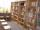The school library contains 6300 books. With this constitutes 7% of professional books for teachers 18% interest books and encyclopedias for pupils and the rest of the fairy tales. How much are which books?
• Of the 2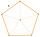Of the shapes,1/6 are triangles, and 5/12 are pentagons. What fraction of the shapes are either triangles or pentagons?
• Ratio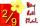Write the ratio with other numbers so that the value is the same: 2: 9
• Numbers divisionWith what number should be divided mixed number 2 3/4 to get 11/12?
• Supermarket 2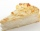A supermarket had a buko pie sale. In the morning, 2/3 of the pies were sold, and in the afternoon, 1/6 of the pies were sold. If 150 pies were left, how many pies had been sold? Show your solution.
• Max vs SharonMax is 1 1/2 times as tall as Sharon. Alan is 4 1/3 feet tall. If Alan is 6 inches taller than Sharon, how tall is Max?
• The Mayflower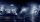The Mayflower traveled for 66 days on the trip from England to America. The weather was storming for many days of their trip. If one and a half of the days at Sea where Sunny with good weather, 1/6 of the days were sunny but very windy and the other days
• Swimming pool 5A rectangular inflatable swimming pool is 3 yards long, 2 5/8 yards wide, and  1 3/5 yard tall. What is the volume of the pool? Round to the nearest tenth.
• Janna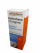Janna takes her medicine 3 times a day. How many days will a 60 ml medicine last if 2 1/2ml is taken each time?
• Three friendsCuba, Matthew and their friend Adam found a brigade during their weekend weekends because they wanted to make a joint trip to the Alps that they planned for the spring break. Cuba enjoyed the skiing trip very much, so he was not lazy to get up and went to